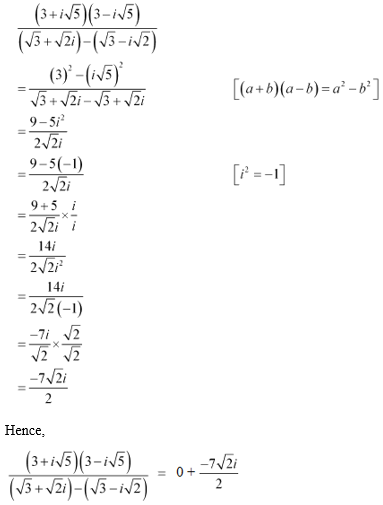# NCERT Solutions For Class 11 Chapter – 5 Exercise – 5.1

NCERT Solutions For Class 11 Math Chapter – 5 Exercise – 5.1

Express each of the complex number given in the Exercises 1 to 10 in the form a + ib.

1. (5i) (-3/5i)

Solution:

(5i) (-3/5i) = 5 x (-3/5) x i2

= -3 x -1 [i2 = -1]

= 3

Hence,

(5i) (-3/5i) = 3 + i0

2. i9 + i19

Solution:

i9 + i19 = (i2)4. i + (i2)9. i

= (-1)4 . i + (-1)9 .i

= 1 x i + -1 x i

= i – i

= 0

Hence,

i9 + i19 = 0 + i0

3. i-39

Solution:

i-39 = 1/ i39 = 1/ i4 x 9 + 3 = 1/ (19 x i3) = 1/ i3 = 1/ (-i) [i4 = 1, i3 =

-I and i2 = -1]

Now, multiplying the numerator and denominator by i we get

i-39 = 1 x i / (-i x i)

= i/ 1 = i

Hence,

i-39 = 0 + i

4. 3(7 + i7) + i(7 + i7)

Solution:

3(7 + i7) + i(7 + i7) = 21 + i21 + i7 + i7

= 21 + i28 – 7 [i2 = -1]

= 14 + i28

Hence,

3(7 + i7) + i(7 + i7) = 14 + i28

5. (1 – i) – (–1 + i6)

Solution:

(1 – i) – (–1 + i6) = 1 – i + 1 – i6

= 2 – i7

Hence,

(1 – i) – (–1 + i6) = 2 – i7

6.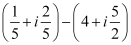Solution: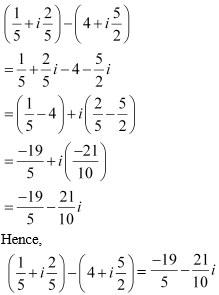7.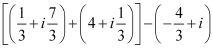Solution: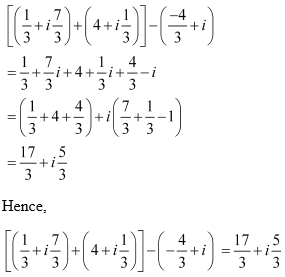8. (1 – i)4

Solution:

(1 – i)= [(1 – i)2]2

= [1 + i2 – 2i]2

= [1 – 1 – 2i]2 [i= -1]

= (-2i)2

= 4(-1)

= -4

Hence, (1 – i)4 = -4 + 0i

9. (1/3 + 3i)3

Solution: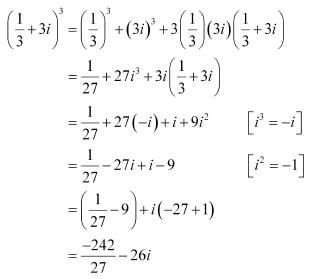Hence, (1/3 + 3i)3 = -242/27 – 26i

10. (-2 – 1/3i)3

Solution: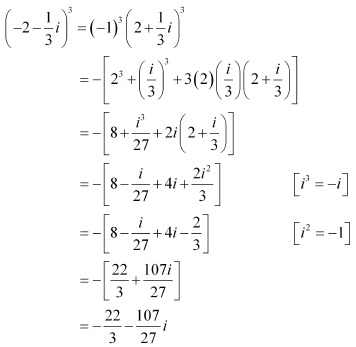Hence,

(-2 – 1/3i)3 = -22/3 – 107/27iFind the multiplicative inverse of each of the complex numbers given in the Exercises 11 to 13.

11. 4 – 3i

Solution:

Let’s consider z = 4 – 3i

Then,

= 4 + 3and

|z|2 = 42 + (-3)2 = 16 + 9 = 25

Thus, the multiplicative inverse of 4 – 3i is given by z-1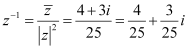12. √5 + 3i

Solution:

Let’s consider z = √5 + 3i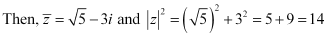|z|2 = (√5)2 + 32 = 5 + 9 = 14

Thus, the multiplicative inverse of √5 + 3i is given by z-1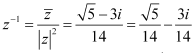13. – i

Solution:

Let’s consider z = –i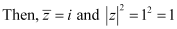Thus, the multiplicative inverse of –i is given by z-1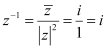14. Express the following expression in the form of a + ib: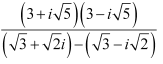Solution: